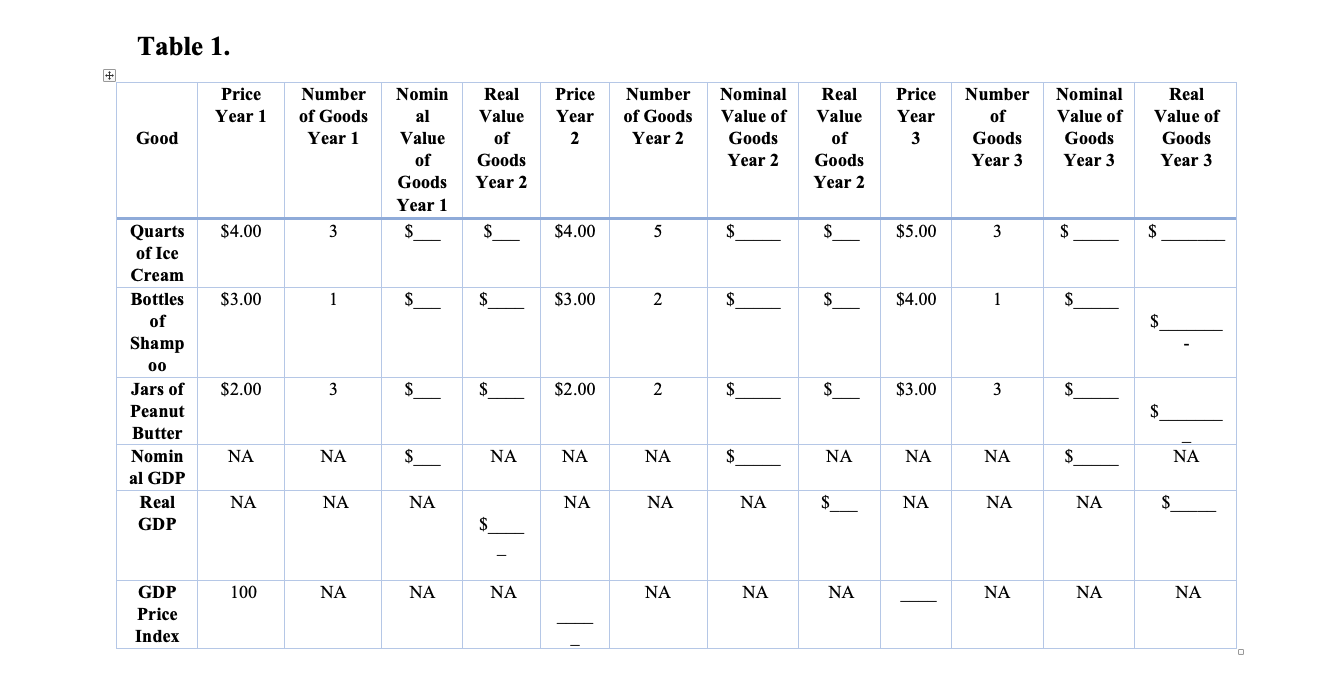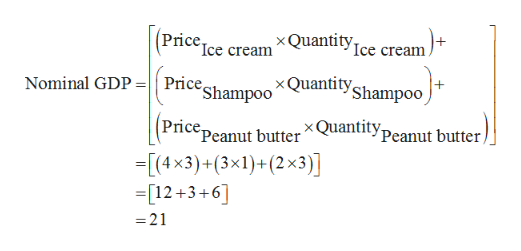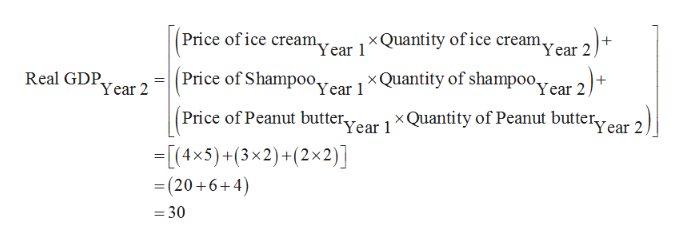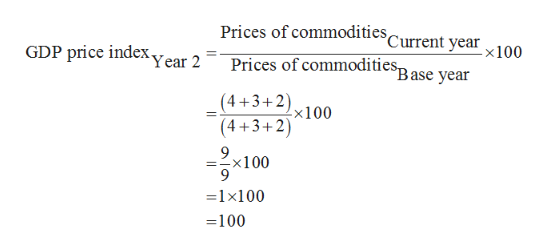# Table 1RealPricePriceNumberNominPriceNumberNominalRealNumberNominalRealValue ofValue ofof GoodsValue ofYear 1of GoodsalValueYearValueYearofGoodsGoodYear 1Valueof2Year 2Goodsof3GoodsGoodsGoodsGoodsYear 3Year 3ofYear 2Year 3GoodsYear 2Year 2Year 1\$5.00Quarts\$4.00\$4.00\$\$353of IceCreamBottles\$3.00\$3.002\$\$\$4.00\$11ofShamp00Jars of\$2.00\$\$2.00\$\$3.0032\$PeanutButterNANA\$NANominNANANANANANAal GDP\$RealNANANANANANANANANA\$GDPGDP100NANANANANANANANANAPriceIndex

Question

Suppose that annual output in year 1 in a 3-good economy is 3 quarts of ice cream, 1 bottle of shampoo, and 3 jars of peanut butter. In year 2, the output mix changes to 5 quarts of ice cream, 2 bottles of shampoo, and 2 jars of peanut butter.

If the prices in both years are \$4 per quart for ice cream, \$3 per bottle of shampoo, and \$2 per jar of peanut butter, what was the economy’s nominal GDP in year 1?

Year 1 - the output combination is - 3 quarts of ice cream, 1 bottle of shampoo, and 3 jars of peanut butter.

Year 2, the output combination changes to 5 quarts of ice cream, 2 bottles of shampoo, and 2 jars of peanut butter.

The prices in both years are \$4 per quart for ice cream, \$3 per bottle of shampoo, and \$2 per jar of peanut butter

Therefore, GDP in year 1 was \$21 [= (3 x \$4) + (1 x \$3) + (3 x \$2)].

Recall that GDP is the core measure of an economy's health. Nominal GDP (also known as current–dollar economic statistics) is not adjusted to account for any price changes. To calculate nominal GDP (the value of all final goods and services evaluated at current-year prices) you have to use the formula: Nominal GDP= P*Q.

To get a real picture of a nation's economic growth economists prefer using real GDP. To calculate real GDP (the value of all final goods and services evaluated at base-year prices for each year) you have to use the formula: Real GDP= P*Q.

In this case, you have to follow a several steps. The first step is to find the value of each good consumed. The second step is to add up the nominal value for the goods for each year separately.

1.2. What was its nominal GDP in year 2? Show the calculation.

Nominal GDP in the second year was \$30 [= (5 x \$4) + (2 x \$3) + (2 x \$2)].

1. Now, assume that in year 3, the output mix changes again to 3 quarts of ice cream, 1 bottles of shampoo, and 3 jars of peanut butter. Consider the year 1 as the base year.

2.1. If the prices in year 3 are \$5 per quart for ice cream, \$4 per bottle of shampoo, and \$3 per jar of peanut butter, what is the economy’s real GDP in year3?

Nominal GDP in the third year was \$28 [= (3 x \$5) + (1 x \$4) + (3 x \$3)].

Deflator = GDP in year 3 / GDP in year 1

= 28/21 = 1.33

Real GDP in year 3 = Nominal GDP/ deflator = 30/1.333 = \$22.5

Question 1

Compute nominal GDP, real GDP, and GDP price index in the year 1 and year 2. Complete the table attached and show the calculation.

Note that the base year is the year where the index is 100.  To calculate GDP price index, you have to divide the price of a collection of goods and services in the specific year (year 2 or year 3) by the price for the same goods and services in a base year (year 1) multiplied by 100. Nominal GDP is then divided by the price index (in hundredths) to determine real GDP

Question 2

What would be the reason (s) of price changes in this a 3-good economy?

Question 3

How would you describe the difference between nominal GDP and real GDPhelp_outlineImage TranscriptioncloseTable 1 Real Price Price Number Nomin Price Number Nominal Real Number Nominal Real Value of Value of of Goods Value of Year 1 of Goods al Value Year Value Year of Goods Good Year 1 Value of 2 Year 2 Goods of 3 Goods Goods Goods Goods Year 3 Year 3 of Year 2 Year 3 Goods Year 2 Year 2 Year 1 \$5.00 Quarts \$4.00 \$4.00 \$ \$ 3 5 3 of Ice Cream Bottles \$3.00 \$3.00 2 \$ \$ \$4.00 \$ 1 1 of Shamp 00 Jars of \$2.00 \$ \$2.00 \$ \$3.00 3 2 \$ Peanut Butter NA NA \$ NA Nomin NA NA NA NA NA NA al GDP \$ Real NA NA NA NA NA NA NA NA NA \$ GDP GDP 100 NA NA NA NA NA NA NA NA NA Price Index fullscreen
check_circle

Step 1

1.
The nominal GDP of the year can be calculated by multiplying the price of a commodity with its quantity and summating the values altogether. In this case there are three commodities such as the ice cream, Shampoo and the peanut butter. The prices of these commodities are \$4, \$3 and \$2 respectively in year 1 and the quantities are 3, 1 and 3 respectively. Thus, the nominal GDP of the year 1 can be calculated by multiplying the prices of each commodity with its quantity and summating them together. It can be calculated as follows:help_outlineImage Transcriptionclosex Quantityce cream. Price Ice cream x Quantity Shampo0 Nominal GDP =Price, Shampoo Price Peanut butter XQuantity peanut butter (4x3)+(3x1)+(2x3)] [12+3+6] 21 fullscreen
Step 2

The nominal GDP of the year 1 is calculated to be \$21. Since the year 1 is the base year for calculating the real GDP of the economy, the real as well as the nominal GDP of the year will be the same. Similarly, the nominal GDP of the year 2 and year 3 can be calculated. The real GDP can be calculated by multiplying the quantity in the year 2 and with the prices of year 1 as follows:help_outlineImage TranscriptionclosePrice of ice creamVear 1 x Quantity of ice creamear 2+ x Quantity of shampooear 2)+ Price of Shampoo, Real GDP Year 2 Year 1 Price of Peanut butter x Quantity of Peanut butterr Year 1 -[(4x5)+(3x2)+(2x2)] = (20+6+4) = 30 fullscreen
Step 3

The real GDP of year 2 is equal to \$30. Similarly, the real GDP of the year 3 can also be calculated. The GDP price index for the year can be calculated by dividing the collection of prices of goo...help_outlineImage TranscriptionclosePrices of commodities, Current year Prices of commodities ase year GDP price indexYear 2 x100 (4+3+2) x100 (4+3+2) -x100 9 1x100 100 fullscreen

### Want to see the full answer?

See Solution

#### Want to see this answer and more?

Solutions are written by subject experts who are available 24/7. Questions are typically answered within 1 hour.*

See Solution
*Response times may vary by subject and question.
Tagged in